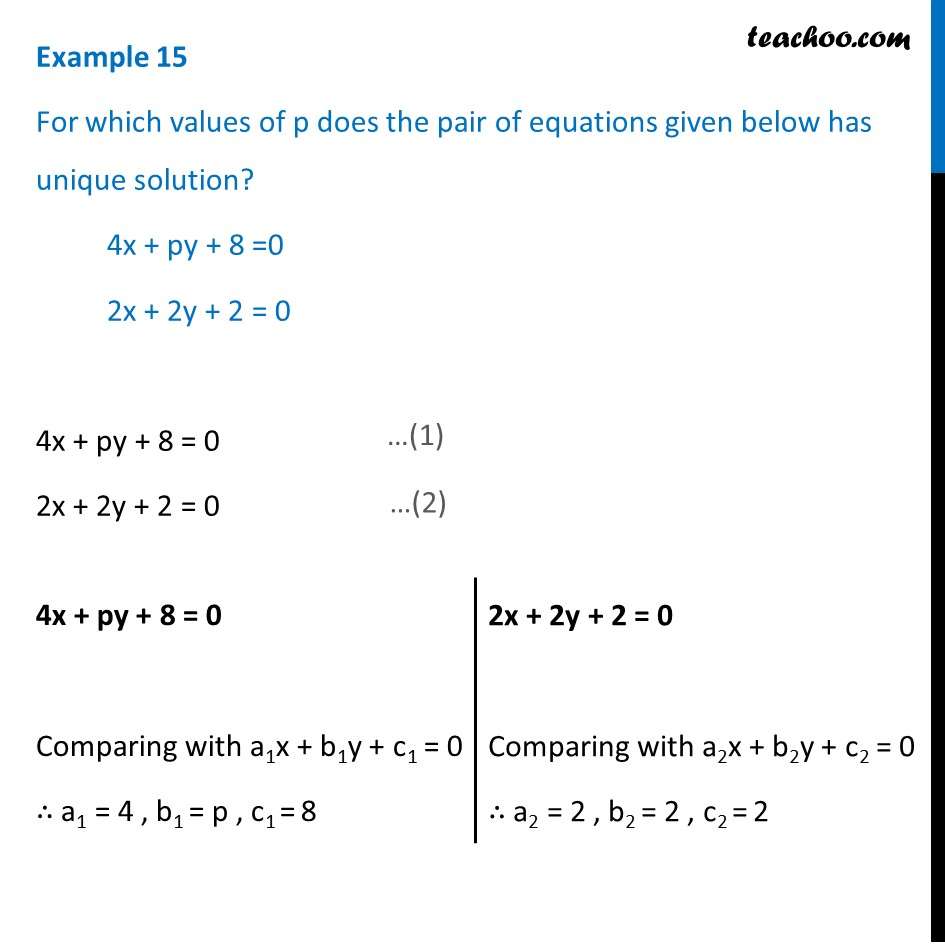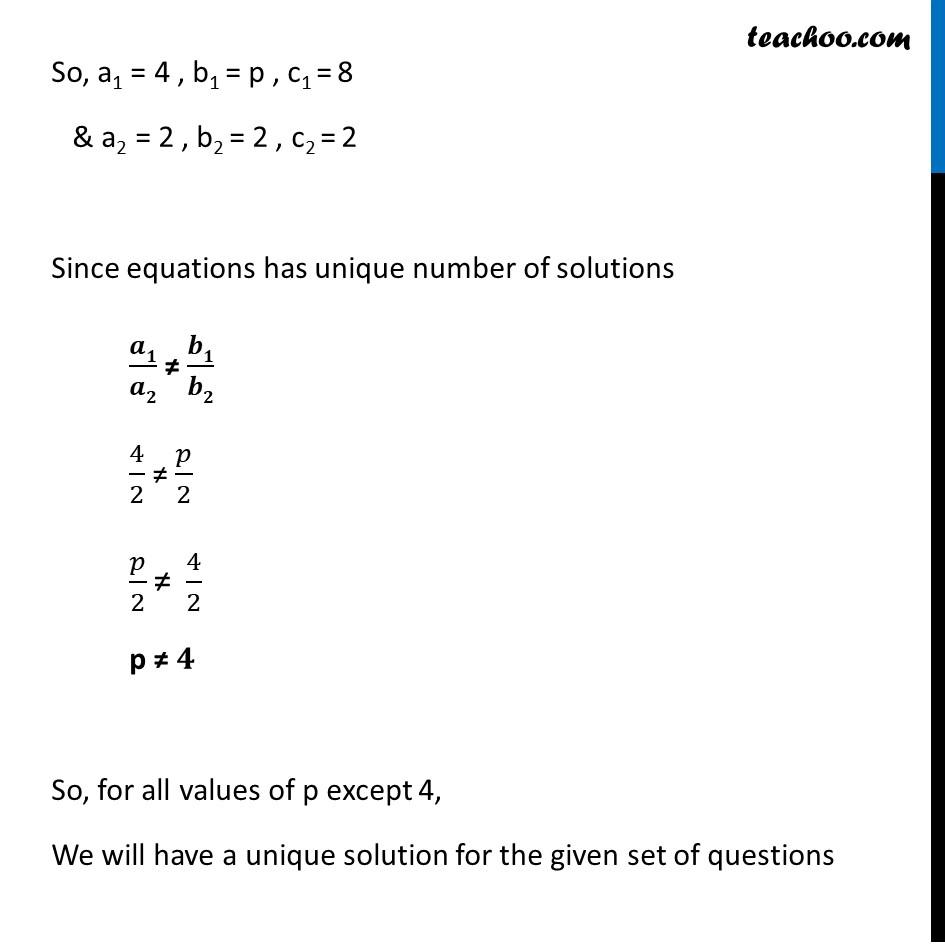1. Chapter 3 Class 10 Pair of Linear Equations in Two Variables (Term 1)
2. Concept wise
3. Cross Multiplication Method

Transcript

Example 15 For which values of p does the pair of equations given below has unique solution? 4x + py + 8 =0 2x + 2y + 2 = 0 4x + py + 8 = 0 2x + 2y + 2 = 0 4x + py + 8 = 0 Comparing with a1x + b1y + c1 = 0 ∴ a1 = 4 , b1 = p , c1 = 8 2x + 2y + 2 = 0 Comparing with a2x + b2y + c2 = 0 ∴ a2 = 2 , b2 = 2 , c2 = 2 So, a1 = 4 , b1 = p , c1 = 8 & a2 = 2 , b2 = 2 , c2 = 2 Since equations has unique number of solutions 𝒂𝟏/𝒂𝟐 ≠ 𝒃𝟏/𝒃𝟐 4/2 ≠ 𝑝/2 𝑝/2 "≠" 4/2 p "≠" 𝟒 So, for all values of p except 4, We will have a unique solution for the given set of questions